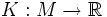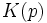# Gaussian curvature

## Definition

### For a regular surface embedded in 3-space

The Gaussian curvature of a regular surface$M$ embedded in$\R^3$ is defined as a map:$K: M \to \R$

given as follows: for$p \in M$,$K(p)$ is the determinant of the shape operator at$p$.

### For an abstract 2-dimensional Riemannian manifold

Fill this in later

### Equivalence of definitions

The equivalence of these definitions arises from Gauss's Theorema Egregium.

## Generalizations

### Generalization of the abstract definition

Further information: sectional curvature,Ricci curvature,scalar curvature

The abstract definition generalizes to the notion of sectional curvature. Sectional curvature is a two-dimensional curvature on manifolds of higher dimension: it takes as input a point on a manifold and a tangent plane at the point, it outputs the Gaussian curvature that we'd get by taking a two-dimensional submanifold with that tangent plane as tangent space.

The Ricci curvature takes a tangent line instead of a tangent plane, and gives the average sectional curvature over all tangent planes containing that tangent line. The scalar curvature averages over all tangent lines.

### Generalization of the concrete definition

Further information: Gauss-Kronecker curvature

The Gauss-Kronecker curvature is valid for codimension one submanifolds (or hypersurfaces) in Euclidean space of any dimension, and uses precisely the same definition as that of the Gaussian curvature.